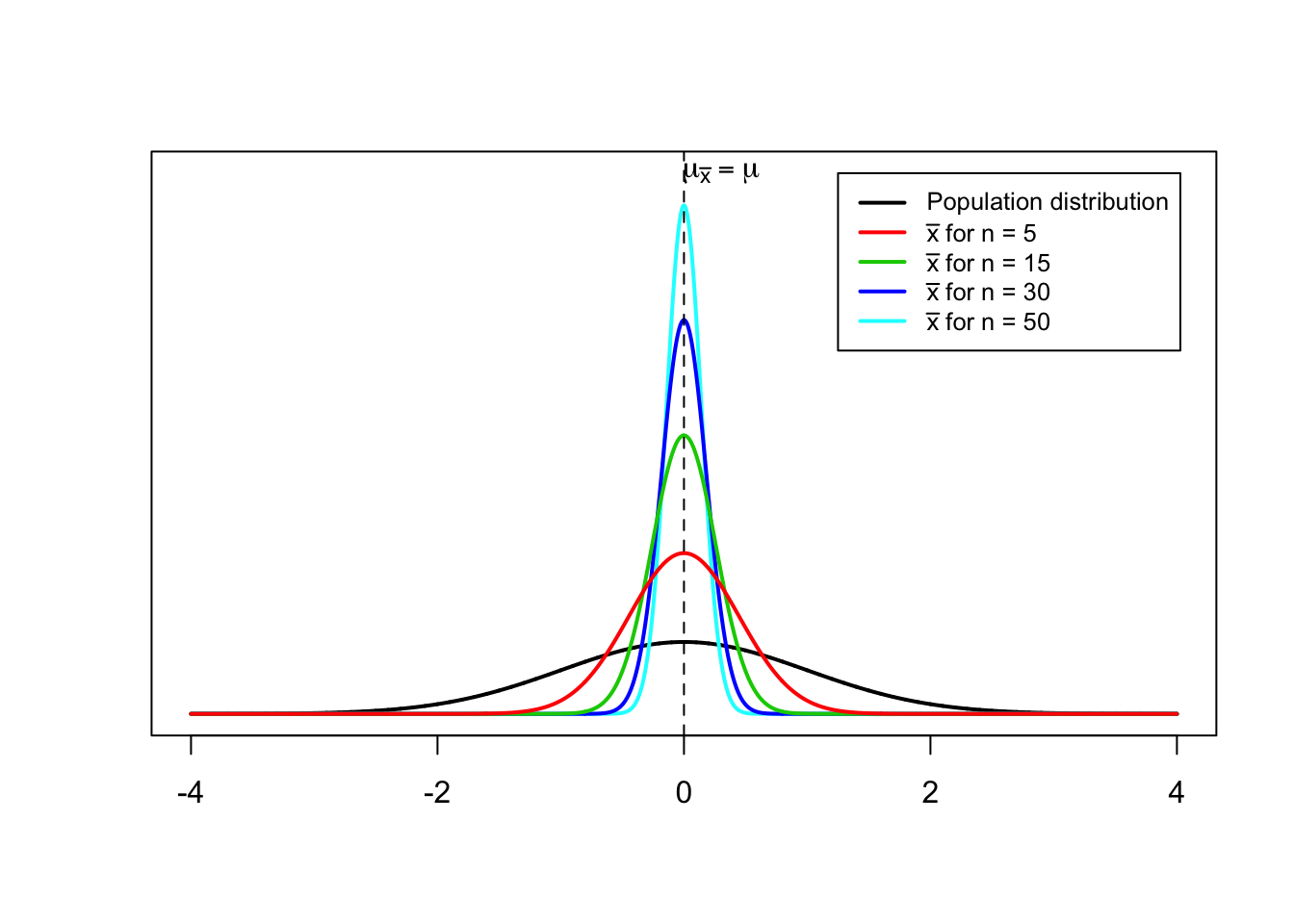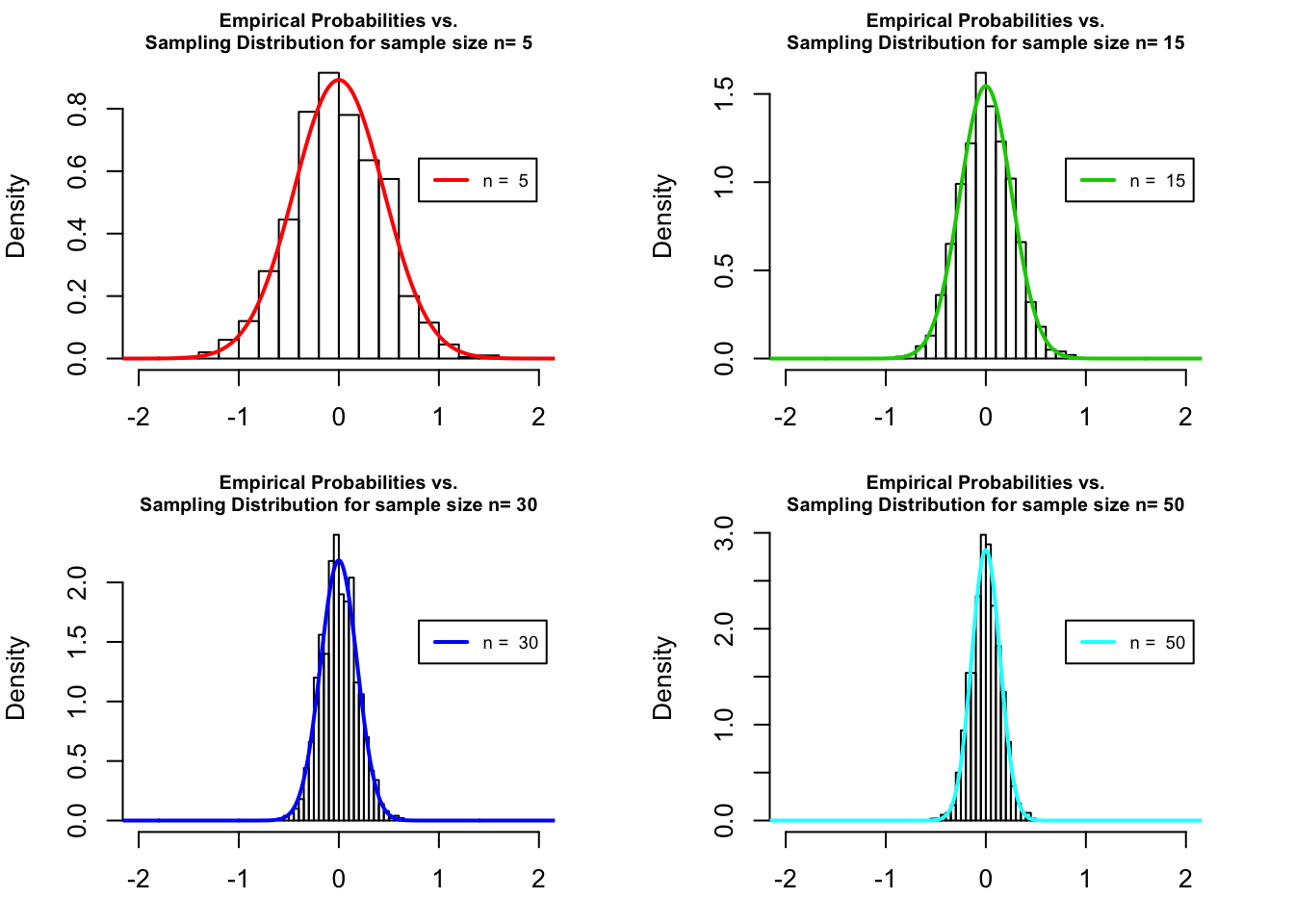#### Shape of the Sampling Distribution

The shape of the sampling distribution relates to the following two cases.
1. The population from which samples are drawn has a normal distribution.
2. The population from which samples are drawn does not have a normal distribution.

#### Sampling from a Normally Distributed Population

When the population from which samples are drawn is normally distributed with its mean equal to $$\mu$$ and standard deviation equal to $$\sigma$$, then:

1. The mean of the sample means, $$\mu_{\bar x}$$, is equal to the mean of the population, $$\mu$$.
2. The standard deviation of the sample means, $$\sigma_{\bar x}$$ is equal to $$\frac{\sigma}{\sqrt{n}}$$, assuming $$\frac{n}{N} \le 0.05$$.
3. The shape of the sampling distribution of the sample means $$(\bar x)$$ is normal, for whatever value of $$n$$.

Let us consider a normally distributed population. For the sake of simplicity we use the standard normal distribution, $$N \sim (\mu, \sigma)$$, with $$\mu = 0$$ and $$\sigma = 1$$. Let us further calculate $$\mu_{\bar x}$$ and $$\sigma_{\bar x}$$ for samples of sample sizes $$n=5,15,30,50$$.

Recall that for a large enough number of repeated sampling $$\mu_{\bar x} \approx \mu$$. Thus, $$\mu_{\bar x}$$ of the different sampling distributions under consideration.

$\mu_{\bar x_{n=5}} = \mu_{\bar x_{n=15}} = \mu_{\bar x_{n=30}} = \mu_{\bar x_{n=50}} = \mu = 0$

Recall the standard error of the sampling distribution $$\sigma_{\bar x} = \frac{\sigma}{\sqrt{n}}$$. Thus, we can easily compute $$\sigma_{\bar x}$$ for $$n=5,15,30,50$$ elements. The different sampling distributions are visualized thereafter.

$\sigma_{\bar x_{n=5}} = \frac{\sigma}{\sqrt{n}} = \frac{1}{\sqrt{5}}\approx 0.447$

$\sigma_{\bar x_{n=15}} = \frac{\sigma}{\sqrt{n}} = \frac{1}{\sqrt{15}}\approx 0.258$

$\sigma_{\bar x_{n=30}} = \frac{\sigma}{\sqrt{n}} = \frac{1}{\sqrt{30}}\approx 0.183$

$\sigma_{\bar x_{n=50}} = \frac{\sigma}{\sqrt{n}} = \frac{1}{\sqrt{50}} \approx 0.141$There are two important observations regarding the sampling distribution of $$\bar x$$

1. The spread of the sampling distribution is smaller than the spread of the corresponding population distribution. In other words, $$\sigma_{\bar x} < \sigma$$.
2. The standard deviation of the sampling distribution decreases as the sample size increases.

In order to verify the 3rd claim from above, that the shape of the sampling distribution of $$\bar x$$ is normal, whatever the value of $$n$$, we conduct a computational experiment. For a large enough number of times (trials = 1000) we sample from the standard normal distribution $$N \sim (\mu =0, \sigma = 1)$$, where each particular sample has a sample size of $$n=5,15,30,50$$. For each sample we calculate the sample mean $$\bar x$$ and visualize the empirical probabilities. Afterwards we compare the empirical distribution of those probabilities with the sampling distributions calculated from the equations above.

trials <- 1000
n <- c(5,15,30,50) #sample size

# emtpy matrix to store results of computations
out <- matrix(nrow = trials, ncol = length(n))

# plotting parameters
my.seq <- seq(-4,4, by=0.001)
color <- c(2,3,4,5,6)

# random sampling
for (i in seq(trials)){
for (j in seq(length(n))) {
out[i,j] <- mean(rnorm(n[j]))
}
}

#plotting
par(mfrow=c(2,2), mar=c(3,4,2,3))

for (i in seq(1,4)){
h <- hist(out[,i],
breaks = 'Scott',
plot = FALSE)
plot(h,
freq = FALSE,
xlim = c(-2,2),
main = paste('Empirical Probabilities vs.\nSampling Distribution for sample size n=', n[i]),
cex.main = 0.75)
curve(dnorm(x,
mean = 0, sd = 1/sqrt(n[i])),
from = -4, to = 4, n=1000,
type = 'l', # set line type
lwd = 2, # set line width
col=color[i]) # set line color
legend(x = 0.8, #set x position
y = max(h\$density)*0.7, #set y position
paste('n = ', n[i]), #set aproriate legend names
lty = 1, # set line type
lwd = 2, # set line width
col = color[i], # set line color
cex = 0.7) # set font size
}The figure verifies the 3rd claim from above: The shape of the sampling distribution of $$\bar x$$ is normal, for whatever value of $$n$$.

In addition, the figure shows that the distribution of the empirical probabilities (bars) fits well the sampling distribution (colored line), and that the standard deviation of the sampling distribution of $$\bar x$$ decreases as the sample size increases. Recall, that the y-axis represents the density, which is a the probability per unit value of the random variable. This is why the probability density can take a value greater than 1, but only over a region with measure less than 1.